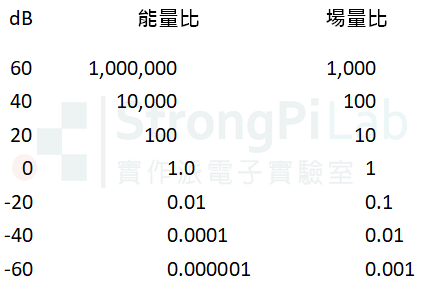# dB 分貝是甚麼-音響與工程上常見的計量方式

• A  每月等距增加1萬元
• B  每月倍數增加2倍

## 自然界只對倍數有感

• 琴鍵上高兩個8度，耳朵只覺得音調”等距”高了2階，但其實頻率已經變為$$2^{2}=4$$倍了
• 地震芮氏規模的每個量級之間，人們只覺得震度固定增加一階，但能量其實差了32倍

## dB 分貝

$$A=10\,log(\frac{P}{P_{ref}})$$ dB   for power quantity

$$A$$為dB數，$$P$$為功率，$$P_{ref}$$為參考功率

$$A=20\,log(\frac{F}{F_{ref}})$$ dB    for field quantity

$$A$$為dB數，$$F$$為場量，$$F_{ref}$$為參考場量## Log前面為何有20

$$power=\frac{V^{2}}{R}=I^{2}R$$  ,  $$V$$=電壓, $$I$$=電流

$A=10\,log(\frac{P}{P_{ref}})=10\,log(\frac{F^{2}}{F_{ref}^{2}})=20\,log(\frac{F}{F_{ref}})$

$$Sound\; Power=\frac{Ap^{2}}{\rho c}cos\theta$$  ,   $$A$$=面積, $$p$$=聲壓,  $$\rho$$=密度,  $$c$$=速度

## 音量的 dB

100 dB的噪音，聲壓並不是$$20\mu Pa$$的100倍喔，而是10萬倍。

## 相關文章

dBm dBmV 是甚麼單位-搞懂dB的衍伸單位